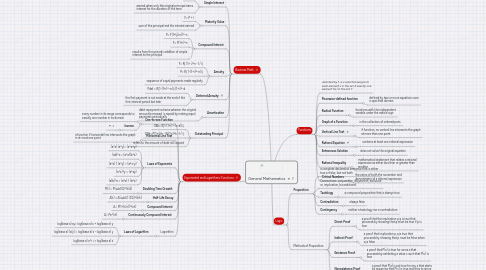# General Mathematics

Get Started. It's FreeGeneral Mathematics## 1. Functions

### 1.2. Piecewise-defined function

1.2.1. defined by two or more equations over a specified domain

1.3.1. functions with the independent variable under the radical sign

### 1.4. Graph of a Function

1.4.1. is the collection of ordered pairs

### 1.5. Vertical Line Test

1.5.1. if function, no vertical line intersects the graph at more than one point

### 1.6. Rational Equation

1.6.1. contains at least one rational expression

### 1.7. Extraneous Solution

1.7.1. does not solve the original equation

### 1.8. Rational Inequality

1.8.1. mathematical statement that relates a rational expression as either less than or greater than another

### 1.9. Critical Numbers

1.9.1. the zeros of both the numerator and denominator of a rational expression

### 2.1. Simple Interest

2.1.1. I = Prt

2.1.2. earned when only the original principal earns interest for the duration of the term

### 2.2. Maturity Value

2.2.1. F= P + I

2.2.2. sum of the principal and the interest earned

### 2.3. Compound Interest

2.3.1. P= F(1+(j/m))^-n

2.3.2. F= P(1+i)^n

2.3.3. results from the periodic addition of simple interest to the principal

### 2.4. Annuity

2.4.1. F= R[ (1+ i)^n -1 / i]

2.4.2. P= R[ 1-(1+i)^-n/i]

2.4.3. sequence of equal payments made regularly

### 2.5. Deferred Annuity

2.5.1. Pdef = R[1-(1+i)^-n/i] (1+i)^-d

2.5.2. the first payment is not made at the end of the first interest period but later

### 2.6. Amortization

2.6.1. debt repayment scheme wherein the original amount borrowed  is repaid by making equal payments periodically

### 2.7. Outstanding Principal

2.7.1. OBk= R[1-(1+i)^-(n-k)/i]

2.7.2. OBk= P(1+i)^k - R[(1+i)^k -1 / i]

2.7.3. refers to the amount of debt still unpaid

## 3. Exponential and Logarithmic Functions

### 3.1. One-to-one Function

3.1.1. every number in its range corresponds to exactly one number in its domain

3.1.2. Inverse

3.1.2.1. f^-1

### 3.2. Horizontal Line Test

3.2.1. a function if horizontal line intersects the graph in at most one point

### 3.3. Laws of Exponents

3.3.1. (a^x) (a^y)= (a^x+y)

3.3.2. (ab)^x = (a^x)(b^x)

3.3.3. (a^x) / (a^y) = (a^x-y)

3.3.4. (a^x)^y = (a^xy)

3.3.5. (a/b)^x = (a^x) / (b^x)

### 3.4. Doubling Time Growth

3.4.1. P(t) = P(sub0)2^(t/d)

### 3.5. Half-Life Decay

3.5.1. A(t) = A(sub0) (1/2)^(t/h)

### 3.6. Compound Interest

3.6.1. A= P(1+(r/n))^(nt)

### 3.7. Continuously Compound Interest

3.7.1. A= Pe^(rt)

### 3.8. Logarithm

3.8.1. Laws of Logarithm

3.8.1.1. log(base a) xy= log(base a) x + log(base a) y

3.8.1.2. log(base a) (x/y) = log(base a) x - log(base a) y

3.8.1.3. log(base a) x^r = r log(base a) x

## 4. Logic

### 4.1. Proposition

4.1.1. a complete declarative sentence that is either true or false, but not both

4.1.2. Connectives: conjunction, disjunction, exclusive or, implication, biconditional

4.1.3. Tautology

4.1.3.1. a compound proposition that is always true

4.1.4.1. always false

4.1.5. Contingency

4.1.5.1. neither a tautology nor a contradiction

### 4.2. Methods of Proposition

4.2.1. Direct Proof

4.2.1.1. a proof that the implication p q is true that proceeds by showing that q must be true if p is true

4.2.2. Indirect Proof

4.2.2.1. a proof that implication p q is true that proceeds by showing that p must be false when q is false

4.2.3. Existence Proof

4.2.3.1. a proof that P(x) is true for some x that proceeds by exhibiting a value c such that P(c) is true

4.2.4. Nonexistence Proof

4.2.4.1. a proof that P(x) is not true for any x that starts by assuming that P(c) is true and tries to arrive at contradiction